## Archive for the 'vibration' Category

29
Jun
07

### Fundamental Modes of Vibration for a Sunset Moth-shaped membrane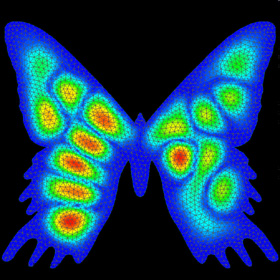Mode displacement plot of the 18th mode of a Sunset Moth-Shaped Membrane. NEiWorks. Just for fun.
Advertisements
27
Jan
06

### Vibrating String with Damping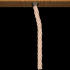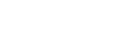This analytical solution for a vibrating string was solved using the method of separation of variables assuming small linear perturbations. The rope was drawn using Mike Williams’ rope macro.

```(* runtime: 2 seconds *) c = 4; L = 1; h = 0.1; b = Pi; k := (n - 0.5)Pi/L; omega := Sqrt[(c k)^2 - (0.5b)^2]; Do[Plot[Evaluate[Sum[(6h/k^2)Sin[k/3] Exp[-0.5b t](Cos[omega t] + (0.5b/omega) Sin[omega t])Sin[k x], {n, 1, 10}]], {x, 0,L}, PlotRange -> {{0, L}, {-h, h}}, AspectRatio -> Automatic], {t, 0, 1.5, 0.025}];```

28
Sep
04

### Vibration Mode of a Spherical Membrane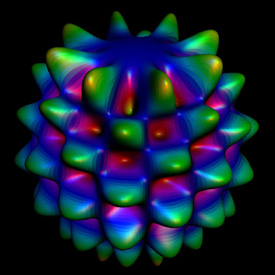Vibration Mode of a Spherical Membrane : This is a somewhat exaggerated example of how a spherical balloon might vibrate.

Vibration Mode of a Spherical MembraneMathematica 4.2, MathGL3d, 9/28/04

```(* runtime: 4 seconds *) Y := Re[SphericalHarmonicY[8, 4, theta, phi]]; r := (1 + 0.5Y); ParametricPlot3D[{r Sin[theta] Cos[phi], r Sin[theta] Sin[phi], r Cos[theta], {EdgeForm[], SurfaceColor[Hue[Y]]}}, {theta, 0, Pi}, {phi, 0, 2Pi}, PlotPoints -> 72, Boxed -> False, Axes -> None]```

21
Apr
04

### Fundamental Modes of Vibration for a Violin-Shaped Membrane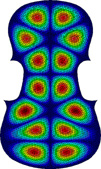Mode displacement plots of the 19th, 28th, and 45th modes, respectively, from left to right, computed with GeoStar 2.8.Click here to see a holographic interferogram of a real violin.
10
Nov
03

### Mode of Vibration of a Circular Membrane31st Fundamental Mode of Vibration of a Circular Membrane. This is an example of how a circular drumhead might vibrate. Click here to see a rotatable animated version.
J3(k43r)cos(3θ) → frequency: f31 = f1k43/k10 = 6.74621 f1

```(* runtime: 5 seconds *) Clear[r]; << NumericalMath`BesselZeros`; k = BesselJZeros[3, 4][]; << MathGL3d`OpenGLViewer`; texture = Graphics[{RGBColor[0, 1, 1], Rectangle[{0, 0}, {10, 10}], RGBColor[0.5, 0, 1], Rectangle[{1, 1}, {9, 9}]}]; MVShow3D[ParametricPlot3D[{r Sin[theta], r Cos[theta], 0.25BesselJ[3, k r]Cos[3 theta]}, {r, 0, 1}, {theta, 0, 2Pi}, PlotPoints -> {56, 168}], MVNewScene -> True, MVTexture -> texture, MVTextureMapType -> MVMeshUVMapping, MVScaleTexture -> {14, 42}] ```

## Welcome !

You will find here some of my favorite hobbies and interests, especially science and art.

I hope you enjoy it!

Subscribe to theRSS feed to stay informed when I publish something new here.

I would love to hear from you! Please feel free to send me an email : bugman123-at-gmail-dot-com

## Recent CommentsBerna Blalack on Magnetic Pendulum Strange…Daan on Magnetic Pendulum Strange…Sebastian Schepis on Diffusion Limited Aggregation…mohammad_andito on CFM56-5 Turbofan Jet Engi…SasQ on Magnetic Field of a Solen… OUPblog » Blog… on Diamond Light Dispersion Complex Roots on Polynomial Roots Joukowski airfoils |… on Joukowski AirfoilKarim Alame on Flapping WingREJISH J on Joukowski Airfoil SOLINOID | Materials… on Magnetic Field of a Solen…Emanuele on 4D “Squarry” Julia…Emanuele on Hydrogen Electron Orbital Prob…Tim on Mandelbrot Set Pickover S…khankasi1 on 4D Quaternion Mandelbrot …

• 561,072 hits
Advertisements